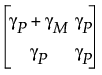Publication date: 08/13/2020

## Statistical Details for the Discrimination Ratio

The discrimination ratio compares the total variance of the measurement, M, with the variance of the measurement error, E. The discrimination ratio is computed for all main effects, including nested main effects. The discrimination ratio, D, is computed as follows:where:

P = estimated variance for a factor

T = estimated total variance